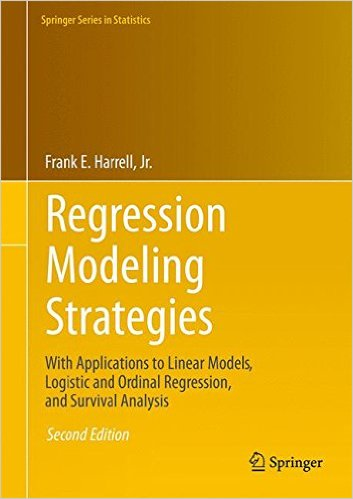[social4i size=”large” align=”float-right”]

Written by Frank E Harrell Jr (first published here)

A major new version of the rms package is now on CRAN.  The most
user-visible changes are:

– interactive plotly graphic methods for model fits.  The best example of
this is survplot for npsurv (Kaplan-Meier) estimates where the number of
risk pop up as you hover over the curves, and you can click to bring up
confidence bands for differences in survival curves

– html methods for model fit summaries especially when using Rmarkdown html
notebooks

– instead of running print(fit, latex=TRUE) use
options(prType=’latex’)
print(fit)
Or: options(prType=’html’)

A complete list of changes is below.  See
http://biostat.mc.vanderbilt.edu/Rrms for an html notebook showing examples
of new features.

Changes in version 5.0-0 (2016-10-31)
* plot.summary.rms: implemented plotly interactive plots if
options(grType=’plotly’) in effect
* plot.anova.rms: implemented plotly interactive plots if
options(grType=’plotly’) in effect; remove psmall argument; changed margin
default to chisq and P
* ggplot.Predict: implemented plotly interactive plots if
options(grType=’plotly’) in effect
* print(fit, md=TRUE), prModFit, prStats: added latex/html methods using
htmlTable package and MathJax for latex math
* html.anova.rms, html.validate, html.summary.rms: new functions for use
with html and MathJax/knitr/RStudio
* latex methods for model fits: added md=TRUE argument to produce
MathJax-compatible latex and html code for fitted models when using R
Markdown
* html: new methods for model fit objects for use with R Markdown
* formatNP: fixed error when digits=NA
* latex.anova.rms: fixed error in not rounding enough columns doe to using
* catg: corrected bug that disallowed explicit catg() in formulas
* ggplot.Predict: added height and width for plotly
* survplot: respected xlim with diffbands.  Thanks: Toni G
* reVector: changed to reListclean and stored model stats components as
lists so can handle mixture of numeric and character, e.g., name of
clustering variable
* survplotp.npsurv: new function for interactive survival curve graphs
using plotly
* anova, summary, latex, print for model fits: use options(grType=’html’)
or ‘latex’ to set output type, output htmltools::HTML marked html so that
* latex methods – set file default to ”
* GiniMd: moved to Hmisc package
* plot.nomogram: fixed bug where abbreviations were being ignored.  Thanks:
Zongheng Zhang
* nomogram: improved examples in help file
* survplot.npsurv: fixed n.risk when competing risks
* survest.cph, survfit.cph, others: fixed large problems due to
incompatibility with survival 2.39-5 for survival predictions; changed
object Strata to strata in cph to be compatible with survival package
* new test survest.r to more comprehensively check survfit.cph and
survest.cph
* ggplot.Predict: quit ignoring xlim; suppress confidence bands if two
group bariables because ggplot2 geom_ribbon doesn’t support multiple
aesthetics
* predictrms: set contrasts for ordered factors to contr.treatment instead
of contr.poly
* val.prob: changed line of identity to use wide grayscale line instead of
dashed line

——————————
Frank E Harrell Jr      Professor and Chairman      School of Medicine

Department of *Biostatistics*      *Vanderbilt University*